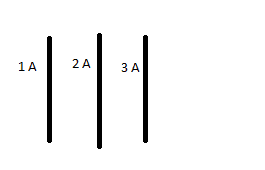# Three conductors, how is the middle one affected

## Homework StatementWe have three equally long conductors with equali distance between each. I have simply drawn it badly. The difference is their currents, they all go in the same direction. How is the middle one effected?

- It goes to the right
- It goes to the left
- It goes up from screen
- It goes down the screen
- Nothing happens

## Homework Equations

B = k * (I/a) and F = I L B

## The Attempt at a Solution

From the above equations we get the formula F = k * I(1) * I(2) * l (length) split by a (distance between two conductors).

What this means is that the force that the conductor with a current of 3A exerts on the middle one is the same as the middle conductor exerts on 3A. Through the use of the right hand rule (http://figures.boundless.com/14036/full/figure-23-04-01a.jpe) we'd see that the middle conductor and the conductor to the right are attracted and the left conductor and the middle conductor are also attracted.

Now, since the attraction to the right is double the size, that will surely overcome the one on the left and therefore the answer is "it goes to the right".

Am I missing something here?